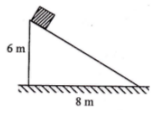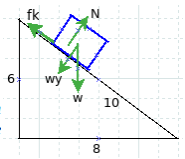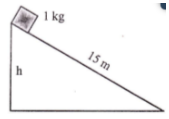# Work-energy principle Nonconservative force Motion on inclined plane with friction – Problems and Solutions

2 Work-energy principle Nonconservative force Motion on inclined plane with friction – Problems and Solutions

1. A block slides down an inclined plane with friction. What is the block’s velocity when the block hits the ground? The coefficient of kinetic friction is 0.4. Acceleration due to gravity is 10 m/s2.

Known :

Initial height (ho) = 6 mFinal height (ht) = 0 m

Initial speed (vo) = 0 (block initially at rest)

Coefficient of kinetic friction (μk) = 0.4

Acceleration due to gravity (g) = 10 m.s-2

cos θ = adj/hyp = 8/10

The vertical component of weight = wy = w cos θ = m g cos θ = m (10)(8/10) = m (10)(4/5) = m (40/5) = 8 m

The normal force = N = wy = 8 m

The force of kinetic friction = fk = μk N = μk wy = (0.4)(8 m) = 3.2 m

Wanted : Final speed (vt)

Solution :

[irp]

The work-mechanical energy principle states that the work done by the nonconservative forces acting on an object is equal to the total change in kinetic and potential energies.

Wnc = ΔEMWnc = ΔEK + ΔEP

Wnc = Work done by nonconservative force, ΔEM = The change in mechanical energy, ΔEK = The change in kinetic energy, ΔEP = The change in potential energy.

The change in kinetic energy :

ΔEK = 1/2 m (vt2 – vo2) = 1/2 m (vt20) = 1/2 m vt2

The change in potential energy :

ΔEP = m g (ht – ho) = m (10)(0-6) = m (10)(-6) = – 60 m

Work done by the force of kinetic friction :

Wnc = – fk s = – (3.2 m)(10) = – 32 m

Minus sign indicates that the work done by the force of kinetic friction on the block is negative.

Determine the final speed (vt) :

Wnc = ΔEK + ΔEP

– 32 m = 1/2 m vt2 – 60 m

– 32 m = m (1/2 vt2 – 60)

– 32 = 1/2 vt2 – 60

– 32 + 60 = 1/2 vt2

28 = 1/2 vt2

2 (28) = vt2

56 = vt2

vt = √4.14

vt = 2√14 m.s-1

[irp]

2.

A block slides down on an inclined plane with friction. The final speed of the block when it hits the ground is 10 m/s2. If the friction force is 2 N and acceleration due to gravity is 10 m/s2. What is the height of h?

Known :

Mass of block (m) = 1 kgInitial speed (vo) = 0 (block initially at rest)

Final speed (vt) = 10 ms-1

Initial height (ho) = h

Final height (ht) = 0

Force of kinetic friction (fk) = 2 N

Acceleration due to gravity (g) = 10 ms-2

Wanted : Height (h)

Solution :

The work is done by the force of kinetic friction :

Wnc = – fk d = – (2)(15) = – 30

Minus sign indicates that the work done by the force of kinetic friction on the block is negative.

The change in kinetic energy :

ΔEK = 1/2 m (vt2 – vo2) = 1/2 (1)(1020) = 1/2 (102) = 1/2 (100) = 50

The change in potential energy :

ΔEP = m g (ht – ho) = (1)(10)(0-h) = (10)(-h) = -10 h

The equation of the work-mechanical energy principle :

Wnc = ΔEK + ΔEP

– 30 = 50 – 10 h

10 h = 50 + 30

10 h = 80

h = 80/10

h = 8 meters

[irp]